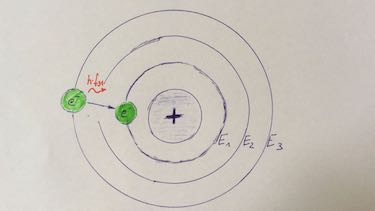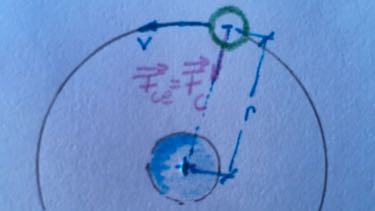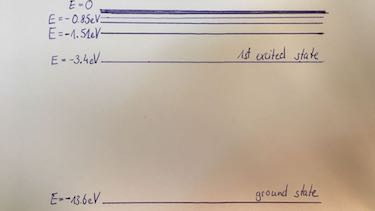An atom is the smallest part of matter. It is so tiny we can't even anymore observe it. Bohr's atomic model explains quantum processes.

1 Bohr's atomic model and postulatesThe Danish physicist Niels Bohr described the atomic model as follows:

1. The positive charged nucleus is orbited by electrons in stationary states with a certain energy level. The quantum conditon: the angular momentum $L$ of a stationary electron is quantised.
$L=m_er_nv_n=\frac{nh}{2\pi } \:\:\: n\in 1,2,...$
2. They can only change their orbit by absorbing or emitting energy in form of electromagnetic radiation. If electrons absorb energy they leave their ground state to excited state.
$\Delta E=hf$
2 energy, radius and velocity for HydrogenEach electron on a orbit $n$, with the distance $r_n$ to the nucleon, has the potential energy $E_{pot}=-\frac{\varepsilon_0 e^2}{r_n}$ and the kinetic energy $E_{kin}=\frac{1}{2}m_ev_n$.

In each orbit of the Hydrogen atom the centripetal force $F_{ce}$ has to be balanced by the Coulomb force $F_C$.
$\frac{m_ev^2}{r}=\frac{e^2}{4\pi \varepsilon_0 r^2}$

With use of the angular momentum we can derive velocity $v_n=\frac{e^2}{2 \varepsilon_0 hn}$ and radius $r_n=\frac{h^2 \varepsilon_0}{\pi m_e e^2}n^2$.

3 energy levelImagine you slip down 3 m and get stuck in a steep sided pit. You lose 2000 J of potential energy and if you cannot gain again 2000 J kinetic energy you got stuck in the pit. This situation is described as potential well.

If you want to ionize an atom by removing an electron you must provide energy for the electron to jump from the ground state to the very top of the potential well.

The lowest level is called ground state. All other states are excited states. While the ground state of hydrogen atom is -13.61 eV, the first excited state has an energy level of -3.4 eV. In order to overcome this energy difference you need an energy of 10.21 eV.

4 Emission spectrum of HydrogenThe emission spectrum of the hydrogen atom is as follows:

1. All downward energy transitions to the ground state belong to the Lyman series, giving out photons in the ultra-violet wavelength region.
2. The Balmer series are transitions to the first excited state. The photons have 4 visible lines in the spectrum.
3. The Paschen series has only lines in the infra-red region.
5 dark lines in emission spectrumIf we examine the continuous sun☀ spectrum we can observe dark lines. The dark lines occur, because the photons are absorbed by colder gases in the outside region of the sun☀.

Even though this photons are remitted, only few of them reach the earth since they are remitted in every direction, thus giving dark lines in the spectrum.

Absorption spectra are very useful since they are telling us about the elements present in the source of radiation.

6 characteristic X-ray spectrum and Franck Hertz experimentIn the X-Ray spectrum electromagnetic radiation comes from differnet energy levels. Transition to the ground state (K-orbit) is named $K_\alpha,\: K_\beta,\: K_\gamma$ radiation. Transition to the first excited state (L-orbit) is named $L_\alpha,\: L_\beta,\: L_\gamma$ radiation. The energy can be calculated from the wavelength by $E=h\cdot c/\lambda$.

In Franck Hertz experiment electrons lose their kinetic energy by inelastic collision with the atoms and electrons of the atoms are lifted to the first excited state. By spontaneous emission of radiation these electrons will change back to ground state. By the next glowing the elctrons have gained again enough energy to leave the ground state, so we have only one energy level in the diagram.

#### Question 1 atomic model

Which statement is correct?

#### Question 2 kinetic and potential energy of electrons in Hydrogen atom

 $m_e$ in kg $9.1\cdot 10^{-31}$ $e$ in As $1.602\cdot 10^{-19}$ $h$ in Js $6.6\cdot 10^{-34}$ $\varepsilon_0$ in As/(Vm) $8.85\cdot 10^{-12}$
1. Calculate the radius of the first three orbits.
2. Calculate the velocity of the electrons on the first three orbits.
3. Calculate the kinetic and potential energy of the first three orbits: ground state, first, second and third excited state.
4. Calculate the ratio between Coulomb force and gravitational force of an electron on the first orbit.
5. Draw the energy levels of the Hydrogen atom.
1. radius $r$ of orbit $n$:
 $n$ 1 2 3 $r$ in 10-10 m $0.529$ $2.117$ $4.763$
2. velocity $v$ of electrons on orbit $n$:
 $n$ 1 2 3 $v$ in 106 m/s $2.188$ $1.094$ $0.729$
3. energy levels:
 orbit number $n$ 1 2 3 $E$ in eV $-13.6$ $-3.4$ $-1.5$
1. Coulomb force: $F_C=$
gravitational force: $F_G=$
ratio: $\frac{F_C}{F_G}=\frac{}{}$
2. energy levels of Hydrogen:#### Question 3 ionisation energy

The ionisation energy of Aluminium is 5.99 eV. Assume the energy level for 1st excited state is 1.99 eV and for 2nd excited state is 1.49 eV.

1. Draw a energy level diagram.
2. Calclulate all possible energies of transition.
3. Decide which transition is in the visible spectrum.### Relax ...

Electron energy level.

#### Question 4 X-ray spectrum

The characteristic X-ray spectrum for different elements is examined.

 element Al Fe Zn $\lambda_{K_\alpha}$ in 10-10m $8.36$ $1.95$ $1.45$
1. Calculate the corresponding energy for the first excited state.
2. Draw a wavelength-intensity spectrum of a sample with these three metals.
1.  element Al Fe Zn $\lambda_{K_\alpha}$ in 10-10m $8.36$ $1.95$ $1.45$ $E$ in keV $1.48$ $6.36$ $8.55$
2.### Terms and phrases

 Niels Bohr Danish physicist who described the atomic model with electrons circulating around the nucleus on certain energy levels. nucleus The central area of an atom, consisting of protons and neutrons. electron transition If atoms absorb enough energy, electrons can change their orbit. ground state lowest energy level in an atom excited state electrons on higher energy levels than ground state ionisation energy energy for an electron to jump from the ground state to the top of potential wall.
 energy level diagram a diagram which shows all possible states and the possible transitions Balmer series Emission spectrum from Hydrogen which show only the transition to the 1st excited states. 4 lines are visible. dark lines in emission spectrum occurs if the emitted spectrum is partly absorbed by the surrounding gas characte­ristic X-ray spectrum Transition to the ground state (K-orbit) is named $K_\alpha,\: K_\beta,\: K_\gamma$ radiation. Transition to the first excited state (L-orbit) is named $L_\alpha,\: L_\beta,\: L_\gamma$ radiation.